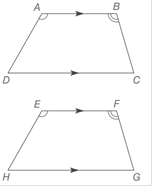Chapter 4.1, Problem 40EElementary Geometry For College St...

7th Edition
Alexander + 2 others
ISBN: 9781337614085

Solutions

Chapter
SectionElementary Geometry For College St...

7th Edition
Alexander + 2 others
ISBN: 9781337614085
Textbook Problem

In quadrilaterals ABCD and EFGH, A B - ∥ D C - , E F - ∥ H G - , ∠ A ≅ ∠ E , and ∠ B ≅ ∠ F , and A D - ≅ E H - .a) Are quadrilaterals ABCD and EFGH congruent?b) Is diagonal D B - congruent to diagonal E G - ?To determine

a.

To find:

The quadrilaterals ABCD and EFGH congruent.

Explanation

Given:

In quadrilaterals ABCD and EFGH, AB-DC-, EF-HG-, AE, and BF, and

Definition:

If quadrilateral ABCD is congruent to quadrilateral EFGH, then all corresponding pairs of angles (A and E ) must be congruent, as the pair of corresponding (AB- and EF-) are congruent

To determine

b.

To find:

The diagonal DB- congruent to diagonal EG- or not.

Still sussing out bartleby?

Check out a sample textbook solution.

See a sample solution

The Solution to Your Study Problems

Bartleby provides explanations to thousands of textbook problems written by our experts, many with advanced degrees!

Get Started

Evaluate the expression sin Exercises 116. 23

Finite Mathematics and Applied Calculus (MindTap Course List)

CHECKPOINT 6. True or false: can be evaluated with formula 1, formula 6, or formula 16.

Mathematical Applications for the Management, Life, and Social Sciences

Sometimes, Always, or Never: fxy = fyx.

Study Guide for Stewart's Multivariable Calculus, 8th

What is the partial fraction form of ?

Study Guide for Stewart's Single Variable Calculus: Early Transcendentals, 8th